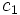# Sufficiently large implies character-separating

## Statement

Let$G$ be a finite group and$k$ a field whose characteristic does not divide the order of$G$. Then, if$k$ is a sufficiently large field for$G$ (that is,$k$ contains all the$m^{th}$ roots of unity where$m$ is the exponent of$G$), then$k$ is a character-separating field for$G$.

By$k$ is character-separating for$G$, we mean that given two distinct conjugacy classes$c_1$ and$c_2$ of$G$ and elements$g_i \in c_i$, there exists a linear representation$\rho$ whose character takes different values on$g_1$ and$g_2$.

## Facts used

• Sufficiently large implies splitting: If$k$ is a sufficiently large field for$G$, then$k$ is a splitting field for$G$: every representation of$G$ over$k$ is completely reducible, and every representation irreducible over$k$ is irreducible over any field extension of$k$.
• Splitting implies character-separating: Any splitting field for a finite group is character-separating: given any two conjugacy classes, there is a linear representation whose character takes different values on these conjugacy classes.